# Atomic mass RELATIVE ATOMIC MASS Ar Mass number

• Slides: 28Atomic mass RELATIVE ATOMIC MASS, Ar (“Mass number”) = number of protons + number of neutrons SYMBOL PROTON NUMBER = number of protons (obviously) Dr Seemal Jelani 1Relative formula mass, Mr The relative formula mass of a compound is the relative atomic masses of all the elements in the compound added together. E. g. water H 2 O: Relative atomic mass of O = 16 Relative atomic mass of H = 1 Therefore Mr for water = 16 + (2 x 1) = 18 Work out Mr for the following compounds: 1) HCl H=1, Cl=35 so Mr = 36 2) Na. OH Na=23, O=16, H=1 so Mr = 40 3) Mg. Cl 2 Mg=24, Cl=35 so Mr = 24+(2 x 35) = 94 4) H 2 SO 4 H=1, S=32, O=16 so Mr = (2 x 1)+32+(4 x 16) = 98 5) K 2 CO 3 K=39, C=12, O=16 so Mr = (2 x 39)+12+(3 x 16) = 138 Dr Seemal Jelani 2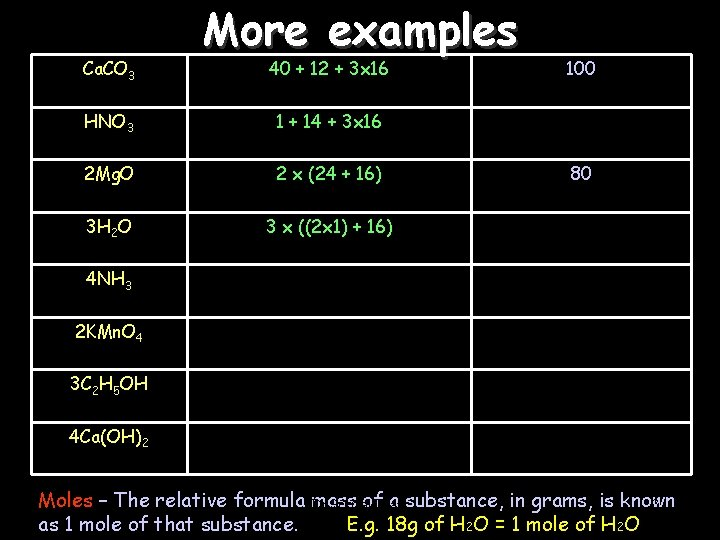Ca. CO 3 More examples 40 + 12 + 3 x 16 HNO 3 1 + 14 + 3 x 16 2 Mg. O 2 x (24 + 16) 3 H 2 O 3 x ((2 x 1) + 16) 100 80 4 NH 3 2 KMn. O 4 3 C 2 H 5 OH 4 Ca(OH)2 Moles – The relative formula mass of. Jelani a substance, in grams, is known Dr Seemal 3 as 1 mole of that substance. E. g. 18 g of H 2 O = 1 mole of H 2 O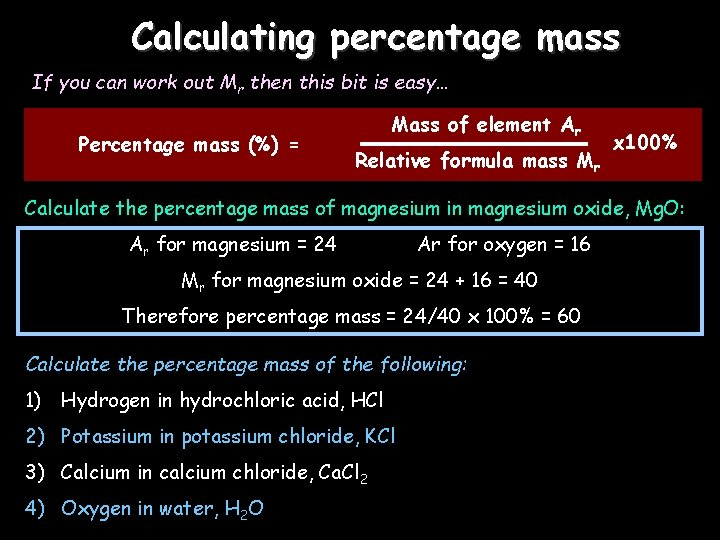Calculating percentage mass If you can work out Mr then this bit is easy… Mass of element Ar Percentage mass (%) = Relative formula mass Mr x 100% Calculate the percentage mass of magnesium in magnesium oxide, Mg. O: Ar for magnesium = 24 Ar for oxygen = 16 Mr for magnesium oxide = 24 + 16 = 40 Therefore percentage mass = 24/40 x 100% = 60% Calculate the percentage mass of the following: 1) Hydrogen in hydrochloric acid, HCl 2) Potassium in potassium chloride, KCl 3) Calcium in calcium chloride, Ca. Cl 2 4) Oxygen in water, H 2 O Dr Seemal Jelani 4Empirical formulae 25/11/2020 Empirical formulae is simply a way of showing how many atoms are in a molecule (like a chemical formula). For example, Ca. O, Ca. CO 3, H 20 and KMn. O 4 are all empirical formulae. Here’s how to work them out: A classic exam question: Find the simplest formula of 2. 24 g of iron reacting with 0. 96 g of oxygen. Step 1: Divide both masses by the relative atomic mass: For iron 2. 24/56 = 0. 04 For oxygen 0. 96/16 = 0. 06 Step 2: Write this as a ratio and simplify: 0. 04: 0. 06 is equivalent to 2: 3 Step 3: Write the formula: Dr Seemal Jelani 2 iron atoms for 3 oxygen atoms means the formula is Fe 2 O 3 5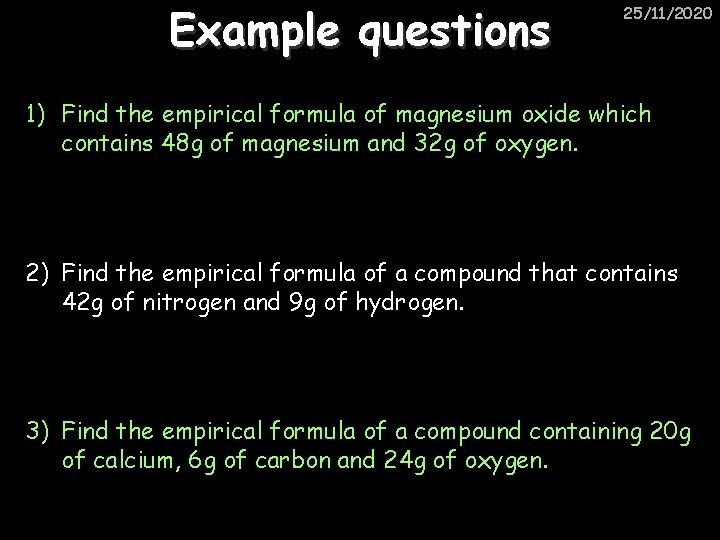Example questions 25/11/2020 1) Find the empirical formula of magnesium oxide which contains 48 g of magnesium and 32 g of oxygen. 2) Find the empirical formula of a compound that contains 42 g of nitrogen and 9 g of hydrogen. 3) Find the empirical formula of a compound containing 20 g of calcium, 6 g of carbon and 24 g of oxygen. Dr Seemal Jelani 6Calculating the mass of a product 25/11/2020 E. g. what mass of magnesium oxide is produced when 60 g of magnesium is burned in air? Step 1: READ the equation: 2 Mg + O 2 2 Mg. O IGNORE the oxygen in step 2 – the question doesn’t ask for it Step 2: WORK OUT the relative formula masses (Mr): 2 Mg = 2 x 24 = 48 2 Mg. O = 2 x (24+16) = 80 Step 3: LEARN and APPLY the following 3 points: 1) 48 g of Mg makes 80 g of Mg. O 2) 1 g of Mg makes 80/48 = 1. 66 g of Mg. O Dr Seemal Jelani 3) 60 g of Mg makes 1. 66 x 60 = 100 g of Mg. O 7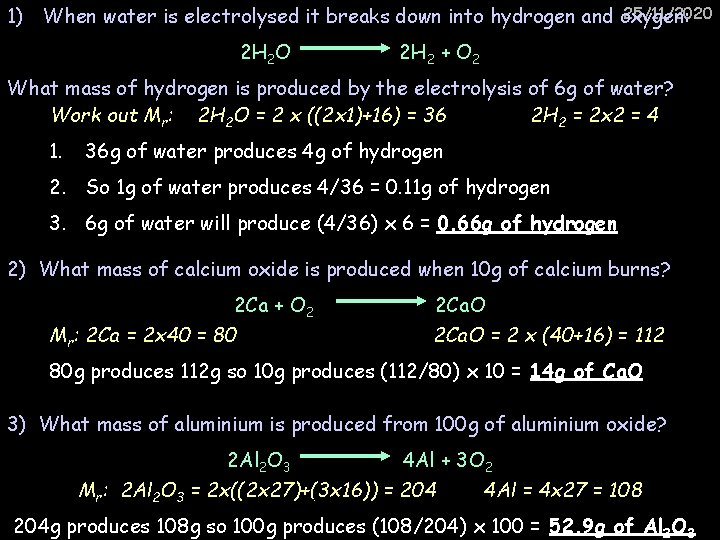25/11/2020 1) When water is electrolysed it breaks down into hydrogen and oxygen: 2 H 2 O 2 H 2 + O 2 What mass of hydrogen is produced by the electrolysis of 6 g of water? Work out Mr: 2 H 2 O = 2 x ((2 x 1)+16) = 36 2 H 2 = 2 x 2 = 4 1. 36 g of water produces 4 g of hydrogen 2. So 1 g of water produces 4/36 = 0. 11 g of hydrogen 3. 6 g of water will produce (4/36) x 6 = 0. 66 g of hydrogen 2) What mass of calcium oxide is produced when 10 g of calcium burns? 2 Ca + O 2 2 Ca. O Mr: 2 Ca = 2 x 40 = 80 2 Ca. O = 2 x (40+16) = 112 80 g produces 112 g so 10 g produces (112/80) x 10 = 14 g of Ca. O 3) What mass of aluminium is produced from 100 g of aluminium oxide? 2 Al 2 O 3 4 Al + 3 O 2 Mr: 2 Al 2 O 3 = 2 x((2 x 27)+(3 x 16)) = 204 Dr Seemal Jelani 4 Al = 4 x 27 = 108 8 204 g produces 108 g so 100 g produces (108/204) x 100 = 52. 9 g of Al 2 O 3Actual Yield 25/11/2020 Even though no atoms are ever gained or lost in a chemical reaction, it is not always possible to obtain the calculated amount of product. Because: • The reaction may not totally finish – it may be reversible • Some of the product may be lost when it is separated from the reaction mixture – filtered • Some of the reactants may react in different ways to the expected reaction Dr Seemal Jelani 9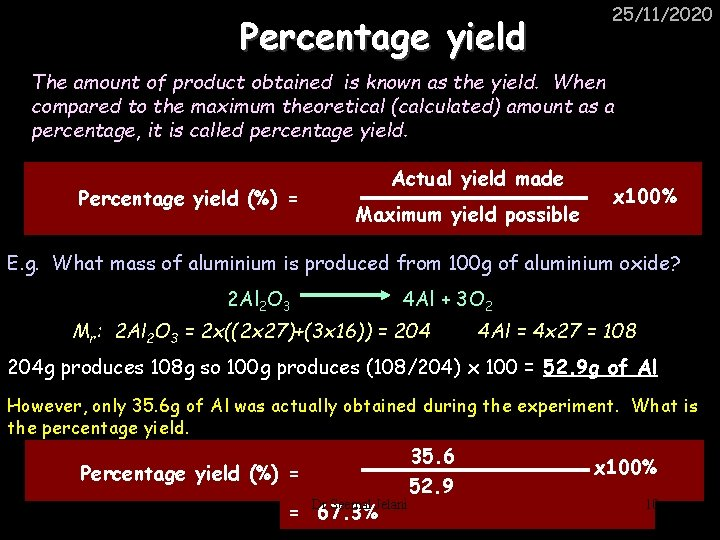Percentage yield 25/11/2020 The amount of product obtained is known as the yield. When compared to the maximum theoretical (calculated) amount as a percentage, it is called percentage yield. Percentage yield (%) = Actual yield made Maximum yield possible x 100% E. g. What mass of aluminium is produced from 100 g of aluminium oxide? 2 Al 2 O 3 4 Al + 3 O 2 Mr: 2 Al 2 O 3 = 2 x((2 x 27)+(3 x 16)) = 204 4 Al = 4 x 27 = 108 204 g produces 108 g so 100 g produces (108/204) x 100 = 52. 9 g of Al However, only 35. 6 g of Al was actually obtained during the experiment. What is the percentage yield. 35. 6 Percentage yield (%) = Dr Seemal Jelani = 67. 3% 52. 9 x 100% 10Mole Ratios A mole ratio converts moles of one compound in a balanced chemical equation into moles of another compound. Dr Seemal Jelani 11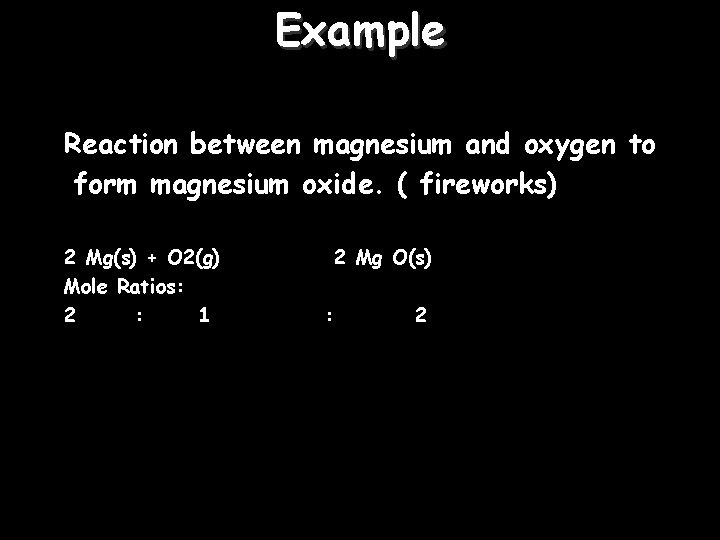Example Reaction between magnesium and oxygen to form magnesium oxide. ( fireworks) 2 Mg(s) + O 2(g) Mole Ratios: 2 : 1 2 Mg O(s) : Dr Seemal Jelani 2 12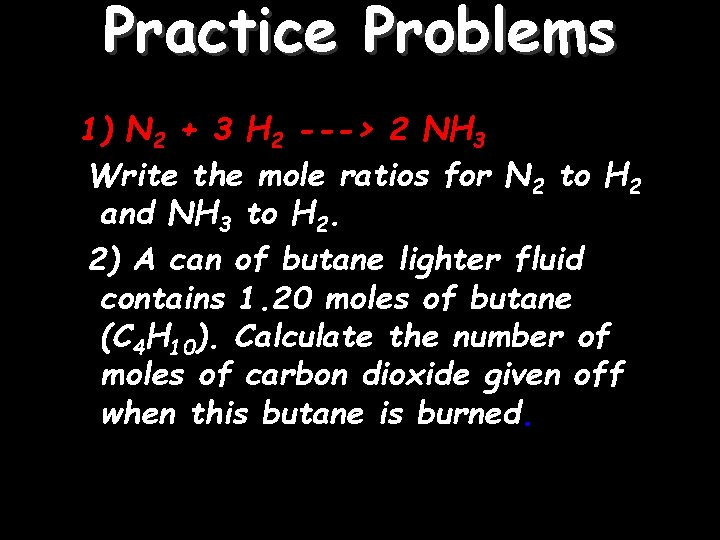Practice Problems 1) N 2 + 3 H 2 ---> 2 NH 3 Write the mole ratios for N 2 to H 2 and NH 3 to H 2. 2) A can of butane lighter fluid contains 1. 20 moles of butane (C 4 H 10). Calculate the number of moles of carbon dioxide given off when this butane is burned. Dr Seemal Jelani 13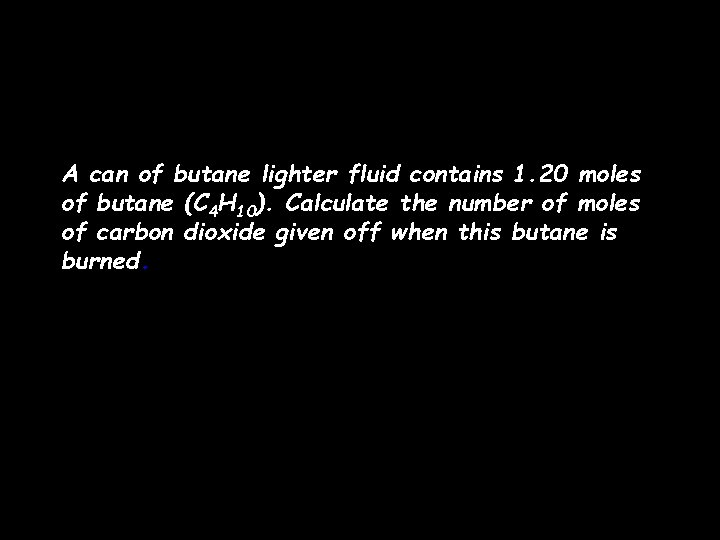A can of butane lighter fluid contains 1. 20 moles of butane (C 4 H 10). Calculate the number of moles of carbon dioxide given off when this butane is burned. Dr Seemal Jelani 14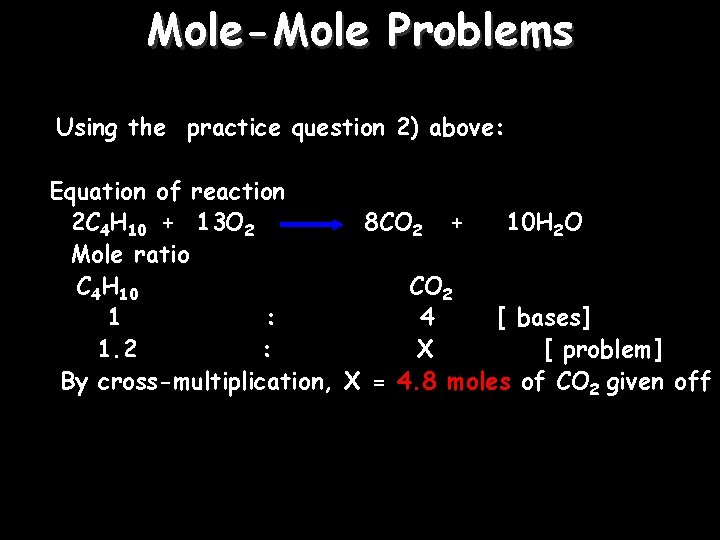Mole-Mole Problems Using the practice question 2) above: Equation of reaction 2 C 4 H 10 + 13 O 2 8 CO 2 + 10 H 2 O Mole ratio C 4 H 10 CO 2 1 : 4 [ bases] 1. 2 : X [ problem] By cross-multiplication, X = 4. 8 moles of CO 2 given off Dr Seemal Jelani 15Mole-Mass Problem 1: 1. 50 moles of KCl. O 3 decomposes. How many grams of O 2 will be produced? [k = 39, Cl = 35. 5, O = 16] 2 KCl. O 3 2 KCl + 3 O 2 Dr Seemal Jelani 16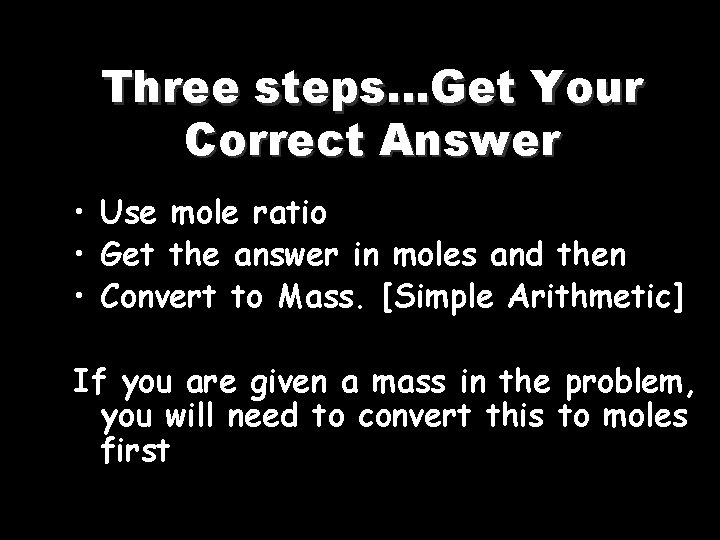Three steps…Get Your Correct Answer • Use mole ratio • Get the answer in moles and then • Convert to Mass. [Simple Arithmetic] If you are given a mass in the problem, you will need to convert this to moles first Dr Seemal Jelani 17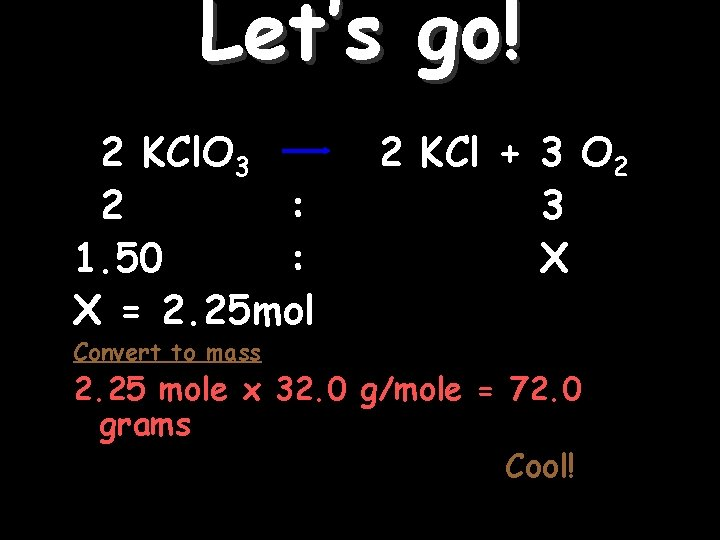Let’s go! 2 KCl. O 3 2 : 1. 50 : X = 2. 25 mol 2 KCl + 3 O 2 3 X Convert to mass 2. 25 mole x 32. 0 g/mole = 72. 0 grams Cool! Dr Seemal Jelani 18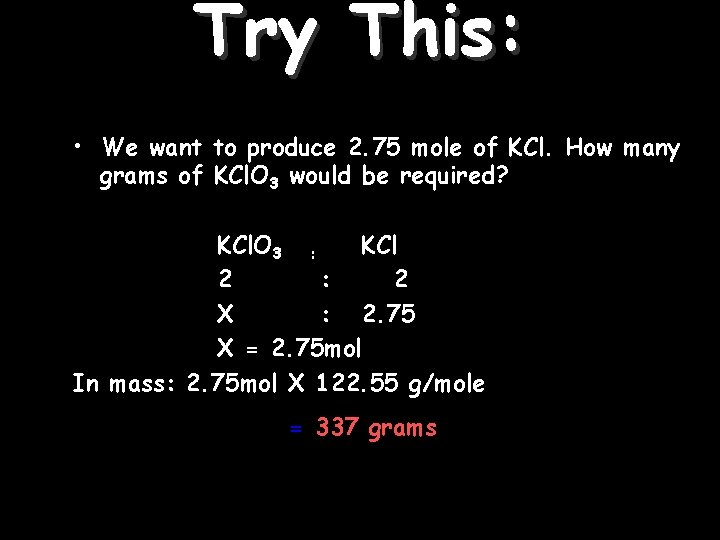Try This: • We want to produce 2. 75 mole of KCl. How many grams of KCl. O 3 would be required? KCl. O 3 : KCl 2 : 2 X : 2. 75 X = 2. 75 mol In mass: 2. 75 mol X 122. 55 g/mole = 337 grams Dr Seemal Jelani 19Conversion of mole to volume No of moles = Volume Molar volume Can you remember a similar equation? Dr Seemal Jelani 20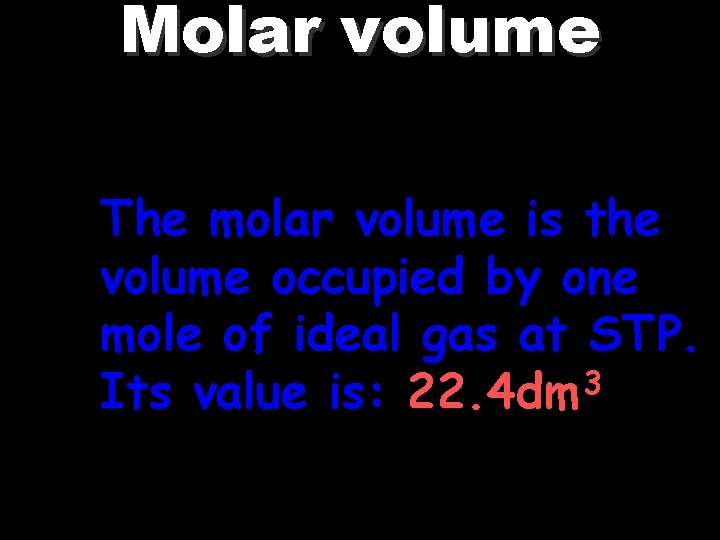Molar volume The molar volume is the volume occupied by one mole of ideal gas at STP. Its value is: 22. 4 dm 3 Dr Seemal Jelani 21Practice Problems Calculate the volume of carbon dioxide formed at STP in ‘dm 3' by the complete thermal decomposition of 3. 125 g of pure calcium carbonate (Relative atomic mass of Ca=40, C=12, O=16) Solution: Convert the mass to mole: Molar mass of Ca. CO 3 = 40 + 12 + (16 x 3) = 100 gmol-1 Mole = mass/molar mass 3. 125/100 = 0. 03125 mol Dr Seemal Jelani 22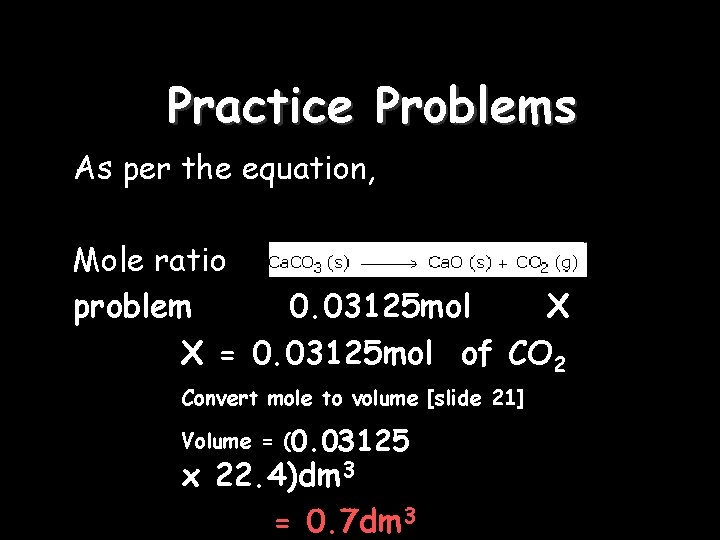Practice Problems As per the equation, Mole ratio 1 : 1 problem 0. 03125 mol X X = 0. 03125 mol of CO 2 Convert mole to volume [slide 21] Volume = (0. 03125 x 22. 4)dm 3 = Dr Seemal Jelani 0. 7 dm 3 23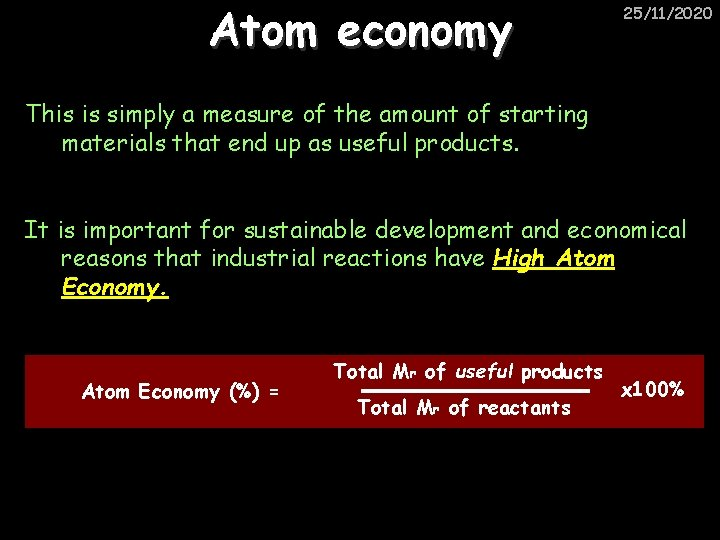Atom economy 25/11/2020 This is simply a measure of the amount of starting materials that end up as useful products. It is important for sustainable development and economical reasons that industrial reactions have High Atom Economy (%) = Total Mr of useful products Total Mr of reactants Dr Seemal Jelani x 100% 24Reversible Reactions 25/11/2020 Some chemical reactions are reversible. In other words, they can go in either direction: A + B e. g. Ammonium chloride NH 4 Cl C + D Ammonia + hydrogen chloride NH 3 + HCl When a reversible reaction occurs in a closed system (Where nothing can escape), equilibrium is reached when both reactions occur at exactly the same rate in each direction. The relative amounts of all the reacting substances at equilibrium depend on the Dr conditions of the reaction. Seemal Jelani 25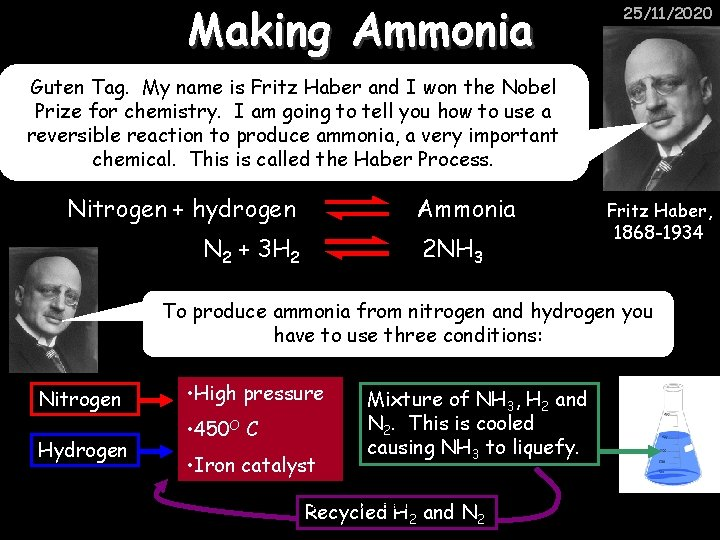Making Ammonia 25/11/2020 Guten Tag. My name is Fritz Haber and I won the Nobel Prize for chemistry. I am going to tell you how to use a reversible reaction to produce ammonia, a very important chemical. This is called the Haber Process. Nitrogen + hydrogen Ammonia N 2 + 3 H 2 2 NH 3 Fritz Haber, 1868 -1934 To produce ammonia from nitrogen and hydrogen you have to use three conditions: Nitrogen Hydrogen • High pressure • 450 O C • Iron catalyst Mixture of NH 3, H 2 and N 2. This is cooled causing NH 3 to liquefy. Dr Seemal Jelani Recycled H 2 and N 2 26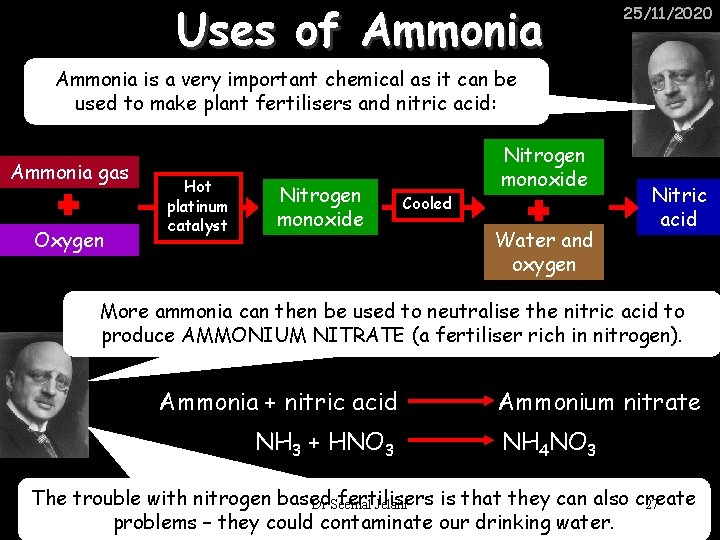Uses of Ammonia 25/11/2020 Ammonia is a very important chemical as it can be used to make plant fertilisers and nitric acid: Ammonia gas Oxygen Hot platinum catalyst Nitrogen monoxide Cooled Nitrogen monoxide Water and oxygen Nitric acid More ammonia can then be used to neutralise the nitric acid to produce AMMONIUM NITRATE (a fertiliser rich in nitrogen). Ammonia + nitric acid NH 3 + HNO 3 Ammonium nitrate NH 4 NO 3 The trouble with nitrogen based fertilisers is that they can also create Dr Seemal Jelani 27 problems – they could contaminate our drinking water.Haber Process Summary 25/11/2020 A low temperature increases the yield of ammonia but is too slow A high temperature improves the rate of reaction but decreases the yield too much A high pressure increases the yield of ammonia but costs a lot of money To compromise all of these factors, these conditions are used to make a reasonable Yield of ammonia, quickly: Nitrogen Hydrogen • 200 atm pressure • 450 O C • Iron catalyst Mixture of NH 3, H 2 and N 2. This is cooled causing NH 3 to liquefy. Dr Seemal Jelani Recycled H 2 and N 2 28